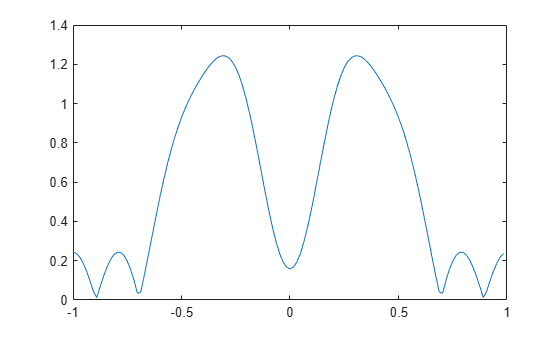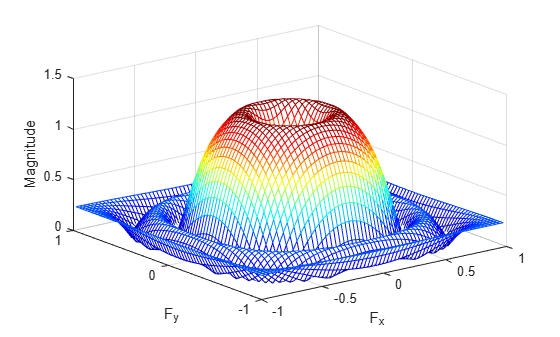ftrans2

2-D FIR filter using frequency transformation

Description

h = ftrans2(b,t) produces the two-dimensional FIR filter h that corresponds to the one-dimensional FIR filter b using the transform t. The transform matrix t contains coefficients that define the frequency transformation to use.

example

h = ftrans2(b) uses the McClellan transform matrix t.

t = [1 2 1; 2 -4 2; 1 2 1]/8;

Examples

collapse all

Use ftrans2 to design an approximately circularly symmetric two-dimensional bandpass filter with passband between 0.1 and 0.6 (normalized frequency, where 1.0 corresponds to half the sampling frequency, or π radians). Since ftrans2 transforms a one-dimensional FIR filter to create a two-dimensional filter, first design a one-dimensional FIR bandpass filter using the Signal Processing Toolbox function firpm.

colormap(jet(64))
b = firpm(10,[0 0.05 0.15 0.55 0.65 1],[0 0 1 1 0 0]);
[H,w] = freqz(b,1,128,'whole');
plot(w/pi-1,fftshift(abs(H)))Use ftrans2 with the default McClellan transformation to create the desired approximately circularly symmetric filter.

h = ftrans2(b);
freqz2(h)Input Arguments

collapse all

FIR filter, specified as a numeric matrix. b must be a 1-D Type I (even symmetric, odd-length) filter such as can be returned by fir1 (Signal Processing Toolbox), fir2 (Signal Processing Toolbox), or firpm (Signal Processing Toolbox).

Data Types: double

The transform matrix, specified as a numeric matrix. t contains coefficients that define the frequency transformation to use. By default, ftrans2 uses a McClellan transform matrix.

Data Types: double

Output Arguments

collapse all

2-D FIR filter, returned as a numeric matrix. ftrans2 returns h as a computational molecule, which is the appropriate form to use with filter2. If t is m-by-n and b has length Q, then h is size ((m-1)*(Q-1)/2+1)-by-((n-1)*(Q-1)/2+1).

Algorithms

The transformation below defines the frequency response of the two-dimensional filter returned by ftrans2.

${{H}_{\left({\omega }_{1},{\omega }_{2}\right)}=B\left(\omega \right)|}_{\mathrm{cos}\omega =T\left({\omega }_{1},{\omega }_{2}\right)},$

where B(ω) is the Fourier transform of the one-dimensional filter b:

$B\left(\omega \right)=\sum _{n=-N}^{N}b\left(n\right){e}^{-j\omega n}$

and T(ω1,ω2) is the Fourier transform of the transformation matrix t:

$T\left({\omega }_{1},{\omega }_{2}\right)=\sum _{{n}_{2}}\sum _{{n}_{1}}t\left({n}_{1},{n}_{2}\right){e}^{-j{\omega }_{1}{n}_{1}}{e}^{-j{\omega }_{2}{n}_{2}}.$

The returned filter h is the inverse Fourier transform of H(ω1,ω2):

$h\left({n}_{1},{n}_{2}\right)=\frac{1}{{\left(2\pi \right)}^{2}}{\int }_{-\pi }^{\pi }{\int }_{-\pi }^{\pi }H\left({\omega }_{1},{\omega }_{2}\right){e}^{j{\omega }_{1}{n}_{1}}{e}^{j{\omega }_{2}{n}_{2}}d{\omega }_{1}d{\omega }_{2}.$

 Lim, Jae S., Two-Dimensional Signal and Image Processing, Englewood Cliffs, NJ, Prentice Hall, 1990, pp. 218-237.